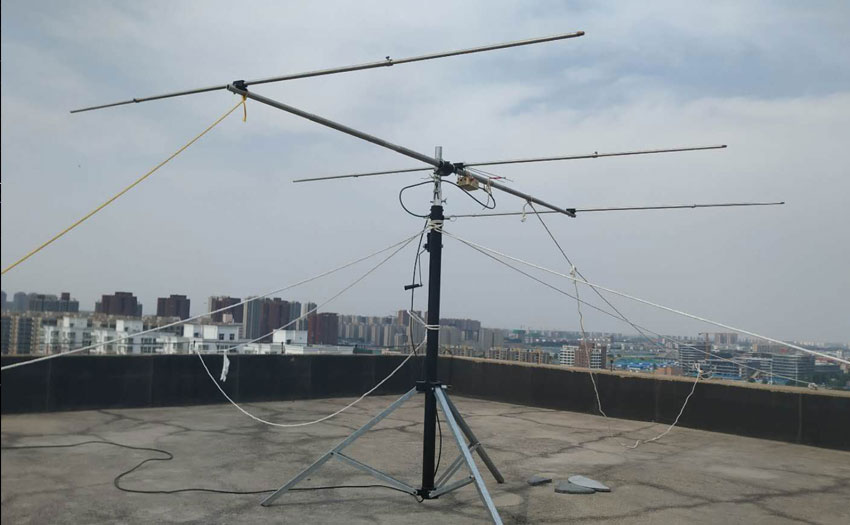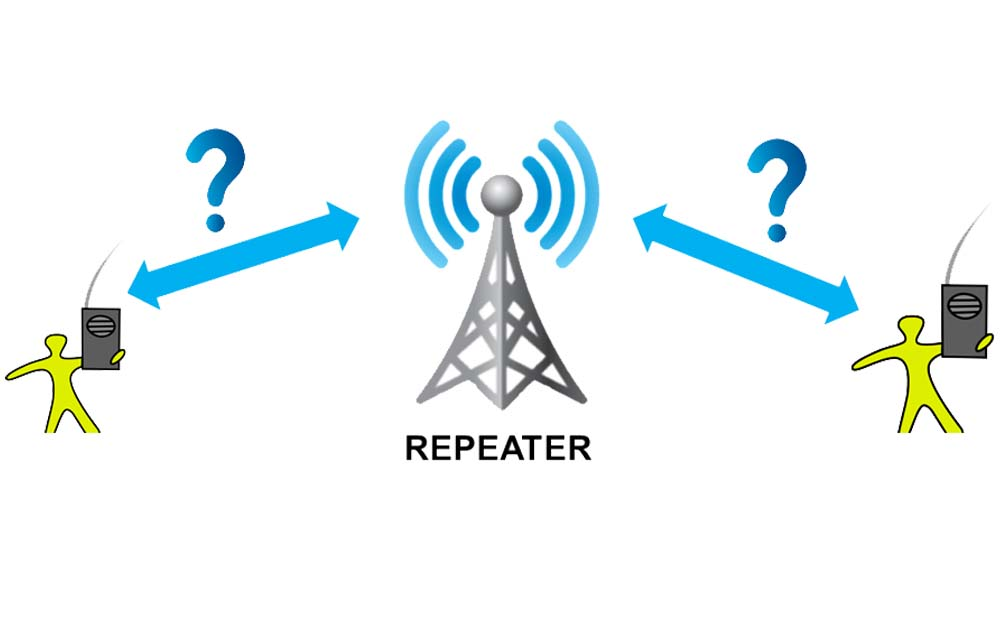# How to estimate the repeater communication distanceDo you know how to calculate the relay communication distance?

For radio system, the repeater( relay station) plays on important role in extending the communication range and coverage. The indispensable equipment for wireless communication system.

For the communication range of repeater, we usually get it from two ways

## 1, Field Test

No matter the handheld radio or repeaters. There are Many external factors can affect field testing, for example: surrounding buildings, the length of the antenna, the distance between the repeater and antenna, the power of your equipment, and etc.

So different geographical conditions have different results on testing the communication range.

## 2, Calculate the range

This is an relatively complicated way to get the communication distance.

Our RT97 is the analog mode repeater, with the power 10w.

## Let’s calculate the communication distance:### First: Get the Transmission loss

Engineering formula for radio wave transmission loss

LM(dB)=88.1+20lgF-20lgh1h2+40lgd

F: The working frequency (mhz)

h1: The antenna height of Communication Object A

h2: The antenna height of Communication Object B

d: The distance between A and B

Note: This formula only applies VHF 150MHZ and UHF 400-470MHZ, and the topographic relief height is about 15m, and the communication distance is within the range of 65km.

### Second: the repeater’s communication range calculate

Assumed conditions

A, Repeater’s working frequency: TX 465MHz RX 455MHz

B, Relay station parameters and erection data

Transmission power: 10W (40dBm)

Receiving sensitivity: -116 DBM

Coaxial cable loss: 2dB

Omnidirectional antenna gain: 9.8dbi

Antenna erection height: 30m

Transmission power: 4W (36dBm)

Receiving sensitivity: -116 DBM

Intercom height: 1.8m

### Third: The system gain between repeater and radio

System gain: the maximum allowable attenuation of the signal transmitted by the radio to the repeater

If feeder cable loss and antenna gain are not taken into account:

System gain (dB) = transmitting power (dBm) – receiving sensitivity (dBm)

If feeder cable loss and antenna gain are taken into account, the system gain in this example is:

SG (dB) =Pt+PA- (RA+CL+RR) =36+0- (9.8-2-116) =144.2 (dB).

In the formula:

RA – repeater antenna gain

CL — coaxial cable loss

### Forth: the system gain with transmission loss formula

If the system gain is equal to the loss of the transmission of the wave, It means that the radio wave energy of the communication distance has reached the limit.

If the system gain is less than the transmission loss, the communication may not be established.

The system gain is substituted into the engineering formula of radio transmission loss

144.2=88.1+20lg455-20lg(1.8*30)+40lgd

144.2=88.1+53.2-34.6+401gd

37.5=40lgd

d=8.7km

## Conclusion:

According to the above parameters, the repeater RT97 has the communication range about 8.7km( Object A to repeater).